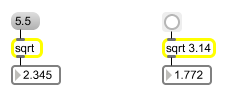sqrt

Square root function

Description

sqrt calculates and outputs the square-root of any given number.

Arguments

Name Type Opt Description
initial-value int or float opt An optional argument specifies the value whose square root is to be output.

Messages

 bang Outputs the currently stored square root value. int input [int] A given number will cause the object to output the square root of that number. A negative input has no real solution, so it causes an output of NaN (Not a Number). float input [float] A given number will cause the object to output the square root of that number. A negative input has no real solution, so it causes an output of NaN (Not a Number).

Output

float: The square root of the input.

Examples